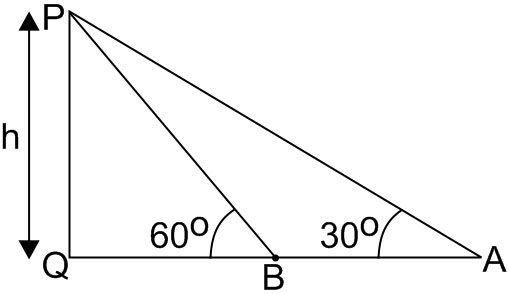Q

# How to solve this problem- A man is walking towards a vertical pillar in a straight path, at a uniform speed. At a certain point A on the path, he observes that the angle of elevation of the top of the pillar is 300. After walking for 10 minutes from

A man is walking towards a vertical pillar in a straight path, at a uniform speed.  At a certain point A on the path, he observes that the angle of elevation of the top of the pillar is 300.  After walking for 10 minutes from A in the same direction, at a point B, he observes that the angle of elevation of the top of the pillar is 600. Then the time taken (in minutes) by him, from B to reach the pillar, is :

• Option 1)

6

• Option 2)

10

• Option 3)

20

• Option 4)

5

295 Views

As we learnt in

Height and Distances -

The height or length of an object or the distance between two distant objects can be determined with the help of trigonometric ratios.

-Let PQ represent pillar. Say height = h

In  ,

In

Now, the person is walking at a uniform speed, hence time taken will be proportional to distance travelled.

Thus,

Hence, time required is 5 minutes.

Option 1)

6

This option is incorrect.

Option 2)

10

This option is incorrect.

Option 3)

20

This option is incorrect.

Option 4)

5

This option is correct.

Exams
Articles
Questions